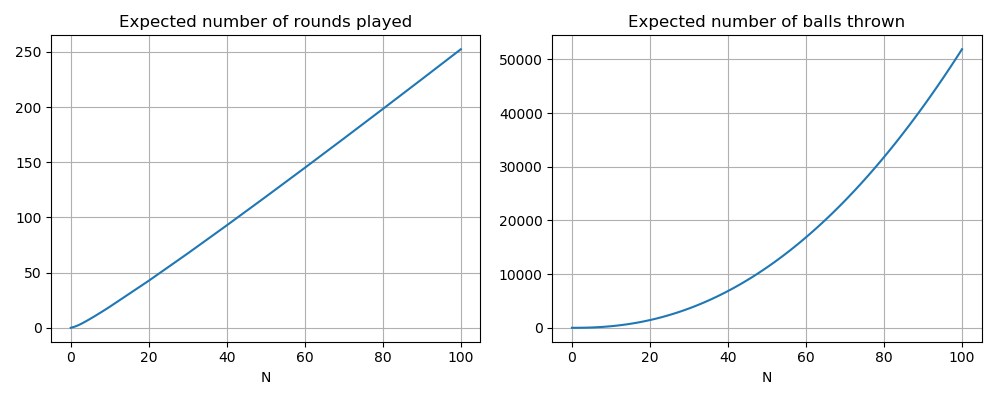# Beer pongThis interesting twist on the game of Beer Pong appeared on the Riddler blog. Here it goes:

The balls are numbered 1 through N. There is also a group of N cups, labeled 1 through N, each of which can hold an unlimited number of ping-pong balls. The game is played in rounds. A round is composed of two phases: throwing and pruning.

During the throwing phase, the player takes balls randomly, one at a time, from the infinite supply and tosses them at the cups. The throwing phase is over when every cup contains at least one ping-pong ball. Next comes the pruning phase. During this phase the player goes through all the balls in each cup and removes any ball whose number does not match the containing cup. Every ball drawn has a uniformly random number, every ball lands in a uniformly random cup, and every throw lands in some cup. The game is over when, after a round is completed, there are no empty cups.

How many rounds would you expect to need to play to finish this game? How many balls would you expect to need to draw and throw to finish this game?

Here is my solution:
[Show Solution]

## 3 thoughts on “Beer pong”

1.Jim Crimmins says:

Hi Laurent:

I did this a bit differently, calculating the expected number of throws in each turn to fill cups using NH_n, with n remaining cups in that turn, then expected cups exhausted in each turn, reducing number of cups, and then proceeding that way, forcing total cups exhausted to be N and total throws to be N^2H_N. These were my reusults curious how close they are to yours:

[1.0, 2.5, 4.166666666666667, 5.916666666666667, 8.3000000000000007, 10.333333333333334, 12.357142857142858, 14.825000000000001, 16.505555555555556, 18.992857142857144]

1.Laurent says:

My results were similar, but different. Here are the numerical results I found:
[1.0, 2.4, 4.17135, 6.09981, 8.12472, 10.2184, 12.3648, 14.5536, 16.7777, 19.0318].
I actually computed exact fractions as well:
$\{1, \tfrac{12}{5}, \tfrac{1485}{356}, \tfrac{317440}{52041 }, \tfrac{7182252125}{883999816}, \tfrac{3139918735968}{307281361145}, \tfrac{2953154676073308334607}{238835679474876862230},\tfrac{1936604564184224758145986176}{133066780411872510357957803},\dots\}$Didn’t include this in the write-up because it wasn’t particularly instructive, but it might be useful to compare actual rational answers. The first major difference is with the 2.4 vs 2.5; that would be a good place to start.

1.Jim Crimmins says:

Tks – it’s not expected to be exact, but it looks pretty close……